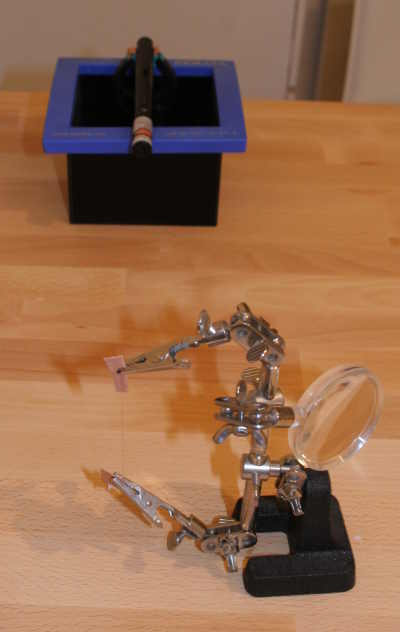You are here: HomePage >> BigScaryLaser >> HairMeasurement

# The width of an hairExperimental setup.
With a laser it is possible to measure the width of very small objects, e.g. of an hair. For this one uses the effect of the diffraction of light on very small objects. In physics they like to use small slits for this type of experiment. The diffraction pattern for complementary objects (like slits and hairs) is identical (Babinet's principle), therefore the same experiment can be made with a hair, which is much easier to acquire.

The image on the right shows one part of the experimental setup. A hair, fixed by two alligator clips, is placed in the path of the laser beam. 10 meters away (9.67m, to be exact), a black surface is used as the screen (because a white screen would reflect too much of the laser light to get a good photograph).

The resulting diffraction pattern is shown in the next photograph:Diffraction pattern.

The distance between two dark spots (minima) is 6.5 cm. The wavelength of the laser is 523 nm.

The explanation of the phenomenon is easier for a slit than for a hair. From the schematic diagram we can derive the formula to calculate the width of the slit [from Ref 1 + 2].Part 1 shows the overall setup. Light rays coming from the left side of the hair need to travel longer to reach the same spot on the right of the screen that rays coming from the right side. Let α be the angle under which we see the first minimum. We can derive the following equation:

```(I)   tan(α) = s/L
```

Actually it should be tan(α) = ( s + d/2 ) / L but as d is small compared with s we can ignore the d/2 part.

Part 2 shows what happens if the difference in the path is exactly half a wavelength (or an uneven multiple of half the wavelength (i.e 0.5, 1.5, 2.5, ...): the two waves cancel each other (the sum of their amplitudes is zero). This happens in the dark spots in the diffraction pattern.

Part 3 explains why the dark spots are where they are. Because the width of the slit is negligibly small compared to the distance hair-screen, we can assume that light rays from the left and right side of the slit that reach the same spot on the screen are parallel. If there is a path difference of λ/2 between the leftmost wave and the light wave from the center of the slit, these two waves cancel each other. The same is true for all waves from the left half of the slit and their conterpart from the right half of the slit (for example the wave from immediately to the left of the center and the rightmost wave, see arrows). There will be a minimum at the correponding spot on the screen. From this we can derive the next equation:

```(II)    sin(α) = λ/d
```

We solve both equations for d:

```(III)  d = λ / sin ( atan(s/L) )
```

With λ = 523*10-9m, s = 6.5*10-2m, L = 9.67m we get d = 7.78*10-5m = 78 micrometers.

This fits well within what Wikipedia tells us about the width of an hair.

For small angles α it is sin(α) = α and tan(α) = α, therefore we can also use these approximate equations:

```(Ia)    α = s/L
(IIa)   α = λ/d
(IIIa)  d = λ*L / s
```

This changes the result only after the fifth decimal place.

References:

1. Arnold Berliner: Lehrbuch der Physik in elementarer Darstellung, 4th Edition, Berlin, 1928.
2. Dr. Rolf Schröder: Slit Diffraction.